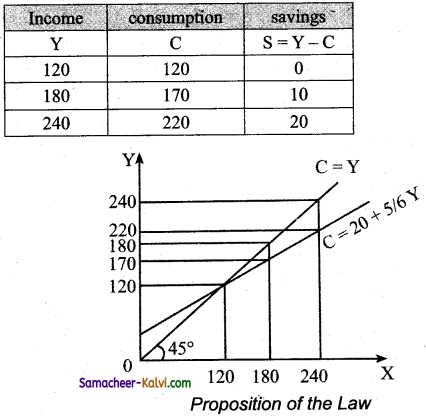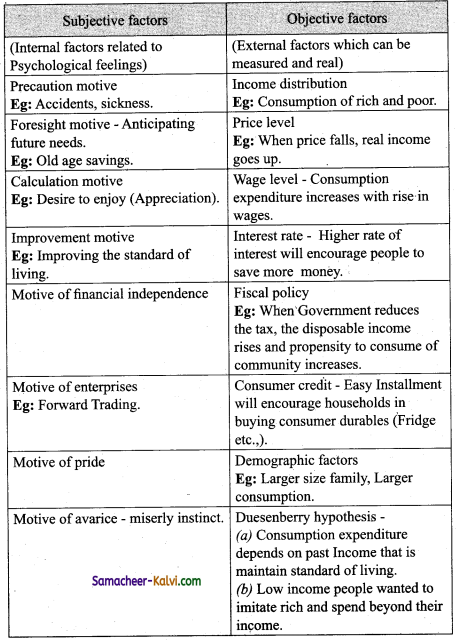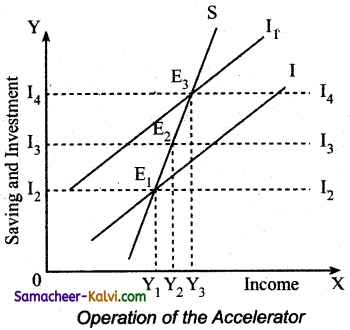## TN State Board 12th Economics Important Questions Chapter 4 Consumption and Investment Functions

Question 1.
What is Consumption function?
The consumption function is the functional relationship between consumption expenditure and the National income = C = f(Y), C = Consumption, Y = Income, f = Function.

Question 2.
What do you mean by Propensity to Consume?
Propensity to consume income and consumption relationship, that is when Income increases, consumption also increases.Question 3.
Define Average Propensity to Consume (APC).
“It is the ratio of consumption expenditure to any particular level of income”.
APC = C / Y
Where C = Consumption and Y = Income

Question 4.
Define Marginal Propensity to Consume (MPC).
“It is the ratio of change in the consumption to change in income”
MPC = ∆C/∆Y
Where ∆C = change in consumption
∆Y = change in income
MPC is positive but less than unity.

Question 5.
What do you mean by Propensity to Save?
It is the proportion of total income or increase in income that consumers save rather than spend on consumption.Question 6.
Define Average Propensity to Save (APS).
APS is the quotient obtained by dividing the total saving by the total income. It is the ratio of total savings to total income. It can be expressed in the form of APS = S/Y Where S = savings Y= income

Question 7.
Define Marginal Propensity to Save (MPS).
It is the ratio of change in saving to a change in income MPS = ∆S / ∆Y
∆S = change in saving
∆Y = change in income
MPC + MPS =1
MPS = 1 – MPC and MPC = 1 – MPS

Question 8.
Define Multiplier.
Multiplier is defined as the ratio of the change in National income to change in income
K = ∆Y / ∆I
Where K = multiplexer
∆I = Increase in investment
∆Y = Resultant increase in incomeQuestion 9.
Define Accelerator.
It is the numerical value of the relation between an increase in consumption and the resulting increase in investment.
Accelerator β = ∆I / ∆C
∆I = change in investment outlays
∆C = change in consumption demand.

Question 10.
State the propositions of Keynes’s Psychological Law of Consumption.

1. When income increases, consumption expenditure also increases but by a smaller amount.
2. The increased income will be divided in some proportion between consumption expenditure and saving.
3. Increase in income always leads to an increase in both consumption and saving.

Question 11.
Differentiate autonomous and induced investment.

 Autonomous investment Induced investment It is independent Planned Income is inelastic Income is elastic Welfare motive Profit motive The curve is horizontal parallel to x axis It is positively slopedQuestion 12.
Explain any three subjective and objective factors influencing the consumption function.
Subjective and Objective factors influencing the consumption function are:

 Subjective factor Objective factor The motive of precaution Income distribution The motive of foresight Price level The motive of calculation Wage level The motive of improvement Interest rate The motive of financial independence Fiscal policy The motive of enterprise Consumer credit The motive of pride Demographic factors The motive of avarice Duesenberry hypothesis

Question 13.
Mention the differences between accelerator and multiplier effect.

 Accelerator Multiplier The numerical value of the relation between an increase in consumption and the resulting increase in investment. It is the ratio of the change in National income to change in investment. Accelerator p = ∆I / ∆C Multiplier K = ∆Y / ∆IQuestion 14.
State the concept of super multiplier.
The super multiplier is worked out by combining both induced consumption and induced investment. It is greater than simple multiplier. It is K and P interaction. J.R.Hicks has combined mathematically K and P and given the name of super multiplier.

Question 15.
Specify the limitations of the multiplier.

1. The assumption of constant capital output ratio is unrealistic.
2. Resources are available only before full employment.
3. Excess capacity is capital goods industries is assumed.
4. Accelerator will work only if the increased demand in permanent.
5. Accelerator will work only when credit is available easily.
6. If there is unused or excess capacity is the consumer goods industry, the accelerator principle would not work.Question 16.
Explain Keynes psychological law of consumption function with diagram.
This law of consumption which forms the basis of the consumption function. This law Implies that there is a tendency on the part of the people to spend in consumption less than the full increment of income.

Assumptions:

1. Ceteris paribus: The other variable like tastes, habits etc., do not change.
2. Existence of normal conditions: The law holds goods under normal conditions.
3. Existence of a Laissez-Faire Capitalist: This law operates in a rich capitalist economy where there is no government interventions.

Propositions to the law:

1. Consumption expenditure increases in a smaller amount when the income increases.
2. The increased income will be divided in some proportion between consumption, expenditure and saving.
3. Consumption and savings increases when income increases.

Three proportions of the law:(i) Proposition (1):
When income increases from 120 to 180, consumption also increases from 120 to 170, but the increase in consumption is less than the increase in income.

(ii) Proposition (2):
When income increases to 180 and 240, it divided between consumption by 170 and 220 by the saving by 10 and 20.

(iii) Proposition(3): Increases in income to 180 and 240 lead to increased consumption 170 and 220 and increased saving 20 and 10 than before.Question 17.
Briefly explain the subjective and objective factors of consumption function.Question 18.
Illustrate the working of Multiplier.
Working of multiplier – Numerical example:
(i) Investment expenditure undertaken by government is ₹ 100cr (on public work). It is from wages prices of material etc.,
(ii) So income of labourers and suppliers of materials increases by ₹ 100 cr.
(iii) If the marginal propensity to consume is 0.8 that is 80%.
(iv) (A sum of ₹ 80cr is spent on consumption and a sum of ₹ 20cr is saved).

On these basis:
(i) Suppliers of goods get an income of ₹ 80cr
(ii) They spent (80% on ₹ 80cr) ₹ 64cr
(iii) The consumption expenditure and income gives a chain reaction.
The final results is ∆Y = 100 + 100 × 4/5 +100 × [4/5]<sup>2</sup> + 100 × [4/5]<sup>3</sup>or,
∆Y = 100+ 100 × 0.8 + 100 × (0.8)<sup>2</sup> + 100 × (0.8)<sup>3</sup>
= 100 + 80 + 64 + 51.2 = 500

that is 100 × 1/1-4/5 100 × 1 / 1 – 4 / 5
100 × 5 = ₹ 500 crores
For instance if C = 100 + 0.8Y, I = 100,
Then Y =100 + 0.8Y+ 100
0.2Y =200
Y = 200 / 0.2= 1000 → Point B
If I is increased to 110, then ,
0.2Y = 210
Y = 210 / 0.2 = 1050 → Point D

For ₹ 10 increase in I, Y has increased by ₹ 50.
This is due to multiplier effect.
At point A, Y = C = 500
C = 100 + 0.8 (500) = 500; S = 0
At point B, Y = 1000
C = 100 + 0.8 (1000) = 900; S = 100 = I
At point D, Y = 1050
C =100 + 0.8 (1050) = 940; S= 110 = I
When I is increased by 10, Y increases by ₹ 50.
This is multiplier effect (K = 5)
K = $$\frac{1}{0.2}$$ = 5Question 19.
Explain the operation of the Accelerator.
(i) To produce 1000 consumer goods 100 machines are required and the life of machine is 10 years, (that is for every 10 years, the machine has to be replaced to maintain to produce 1000 consumer goods). This is called a Replacement Demand.
(ii) If the demand for consumer increases by 10% [ 1000 to 1100] is that case 10 more machines
are needed and the total demand for machines becomes [10 + 10 = 20 ] that is 10 for replacement and 10 for meeting the increased demand.

Note: [The important aspect is 10% increase in demand for consumer goods causes a 100% increase for machines from 10 to 20 No. of machines].

The result derived is:
Even a small change is demand for consumer goods will lead to a big change in investment.Explanation of accelerator:
→ SS is the saving curve(SS).
→ II is the investment curve (II).
→ A is the point E1 the economy is in equilibrium with OY1, income [OY1].
→ Saving and investment are equal at OI2.
→ When investment is increased from OI2 to OI4, the income also increases from OY, to OY3 and the equilibrium point is E3.
→ If the increase in investment by I2I4 is purely exogenous, then the increase in income by Y1 and Y3 would have been due to multiplier effect.
→ According to this diagram, it is assumed that exogeneous investment is only by I2I3 and induced investment is by I3I4.
→ To conclude, the increase in income by Y1 Y3 is due to the multiplier effect and.
→ The increase in income by Y2Y3 is due to the accelerator effect.Question 20.
What are the differences between MEC and MEI?

 Marginal Efliciency of Capital(MEC) Marginal Efficiency of Investment(MEI) It is based on a given supply price for capital. It is based on the induced change in the price due to change in the demand for capital.. It represents the rate of return on all successive units of capital without regard to existing capital. It shows the rate of return on just those units of capital over and above the existing capital stock. The capital stock is taken on the X axis of diagram. The amount of investment is taken on the X – axis of diagram. It is a “stock” concept. It is a “flow” concept. It determines the optimum capital stock in an economy at each level of interest rate. It determines the net investment of the economy at each interest rate given the capital stock.Multiple Choice Questions:

Question 1.
The average propensity to consume is measured by:
(a) C / Y
(b) C × Y
(c) Y / C
(d) C + Y
(a) C / Y

Question 2.
An increase in the marginal propensity to consume will:
(a) Lead to consumption function becoming steeper.
(b) Shift the consumption function upwards.
(c) Shift the consumption function downwards.
(d) Shift savings function upwards.
(a) Lead to consumption function becoming steeper.

Question 3.
If the Keynesian consumption function is C = 10 + 0.8 Y then, if disposable income is ₹ 1000, what is amount of total consumption?
(a) ₹ 0.8
(b) ₹ 800
(c) ₹ 810
(d) ₹ 0.81
(c) ₹ 810Question 4.
If the Keynesian consumption function is C = 10 + 0.8Y then, when disposable income is ₹ 100, what is the marginal propensity to consume?
(a) ₹ 0.8
(b) ₹ 800
(c) ₹ 810
(d) ₹ 0.81
(a) ₹ 0.8

Question 5.
If the Keynesian consumption function is C = 10 + 0.8 Y then, and disposable income is ₹ 100, what is the average propensity to consume?
(a) ₹ 0.8
(b) ₹ 800
(c) ₹ 810
(d) ₹ 0.9
(d) ₹ 0.9

Question 6.
As national income increases:
(a) The APC falls and gets nearer in value to the MPC.
(b) The APC increases and diverges in value from the MPC.
(c) The APC stays constant.
(d) The APC always approaches infinity.
(a) The APC falls and gets nearer in value to the MPC.Question 7.
As increase in consumption at any given level of income is likely to lead:
(a) Higher aggregate demand
(b) An increase in exports
(c) A fall in taxation revenue
(d) A decrease in import spending
(a) Higher aggregate demand

Question 8.
Lower interest rates are likely to:
(a) Decrease in consumption
(b) Increase cost of borrowing
(c) Encourage saving
(d) Increase borrowing and spending
(d) Increase borrowing and spending

Question 9.
The MPC is equal to:
(a) Total spending / total consumption
(b) Total consumption/total income
(c) Change in consumption /change in income
(d) None of the above
(c) Change in consumption /change in incomeQuestion 10.
The relationship between total spending on consumption and the total income is the:
(a) Consumption function
(b) Savings function
(c) Investment function
(d) Aggregate demand function
(a) Consumption function

Question 11.
The sum of the MPC and MPS is:
(a) 1
(b) 2
(c) 0.1
(d) 1.1
(a) 1

Question 12.
As income increases, consumption will:
(a) fall
(b) not change
(c) fluctuate
(d) increase
(d) increaseQuestion 13.
When investment is assumed autonomous the slope of the AD schedule is determined by the:
(a) marginal propensity to invest
(b) disposable income
(c) marginal propensity to consume
(d) average propensity to consume
(c) marginal propensity to consume

Question 14.
The multiplier tells us how much changes after a shift in
(a) Consumption, income
(b) investment, output
(c) savings, investment
(d) output, aggregate demand
(d) output, aggregate demand

Question 15.
The multiplier is calculated as:
(a) 1 / (1- MPC)
(b) 1 / MPS
(c) 1 / MPC
(d) (a) and (b)
(d) (a) and (b)Question 16.
If the MPC is 0.5, the multiplier is:
(a) 2
(b) 1/2
(c) 0.2
(d) 20
(a) 2

Question 17.
In an open economy import ________ the value of the multiplier.
(a) reduces
(b) increase
(c) does not change
(d) changes
(a) reduces

Question 18.
According to Keynes, investment is a function of the MEC and:
(a) Demand
(b) Supply
(c) Income
(d) Rate of interest
(d) Rate of interestQuestion 19.
The term super multiplier was first used by:
(a) J.R.Hicks
(b) R.G.D. Allen
(c) Kahn
(d) Keynes
(a) J.R.Hicks

Question 20.
The term MEC was introduced by:
(b) J.M. Keynes
(c) Ricardo
(d) Malthus
(b) J.M. KeynesSamacheer Kalvi 12th Economics Notes Chapter 4 Consumption and Investment Functions

→ Ceteris paribus: All other things remaining equal.

→ Extraneous: Calculation of the value of a function outside the range of known values.

→ Hyper inflation: Very rapid inflation, monetary authorities will lose control over the situation.

→ Averice: Miserly instinct.

→ Windfall gains or losses: Unexpected changes in the stock market.

→ Investment multiplier: Refers to the multiple increase in income due to a given increase in investment.

→ The minimum value of multiplier: It is one (1) and maximum value is infinity.

→ Ex-ante: Intended or planned

→ Ex-post: Actual or realised

→ Break eveb point: The point at which the volume of sales yields revenue equal to the entire fixed and variable cost. At this point profit is zero.→ CF – Consumption function

→ AC – Autonomous Consumption

→ APC – Average Propensity to Consume

→ APS – Average Propensity to Save

→ MPC – Marginal Propensity to Consume

→ MPS – Marginal Propensity to Save

→ Y – Income, C – Consumption, S – Savings,

→ Investment function:
I = f(R)
I – Investment (dependent variable)
R – Rate of interest (independent variable)

→ MEC – Marginal Efficiency of Capital

→ MEI Marginal Efficiency of Investment→ Consumption function symbolically C = f(Y).
C – Consumption, Y – Income,f- Function

→ Marginal propensity to consume = $$\frac{\Delta \mathrm{C}}{\Delta \mathrm{Y}}$$

→ Average propensity to consume = $$\frac{C}{Y}$$

→ Average propensity to save = $$\frac{\mathrm{S}}{\mathrm{Y}}$$

→ Marginal propensity to save = $$\frac{\Delta \mathrm{S}}{\Delta \mathrm{Y}}$$

→ Multiplier K = $$\frac{\Delta \mathrm{Y}}{\Delta \mathrm{I}}$$ (or)
K = $$\frac{1}{1}$$ – MPC (or) $$\frac{1}{\text { MPS }}$$

→ Accelerator Effect β = $$\frac{\Delta I}{\Delta C}$$

→ Leverage Effect Y = C + IA + Ip

TN Board 12th Economics Important Questions